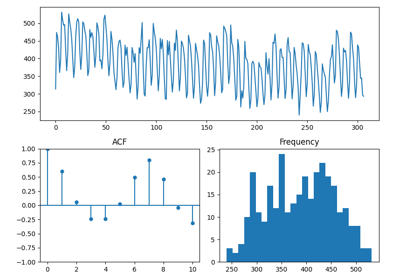# pmdarima.arima.ndiffs¶

pmdarima.arima.ndiffs(x, alpha=0.05, test='kpss', max_d=2, **kwargs)[source][source]

Estimate ARIMA differencing term, d.

Perform a test of stationarity for different levels of d to estimate the number of differences required to make a given time series stationary. Will select the maximum value of d for which the time series is judged stationary by the statistical test.

Parameters: x : array-like, shape=(n_samples, [n_features]) The array (time series) to difference. alpha : float, optional (default=0.05) Level of the test. This is the value above below which the P-value will be deemed significant. test : str, optional (default=’kpss’) Type of unit root test of stationarity to use in order to test the stationarity of the time-series. One of (‘kpss’, ‘adf’, ‘pp’) max_d : int, optional (default=2) Maximum number of non-seasonal differences allowed. Must be a positive integer. The estimated value of d will not exceed max_d. d : int The estimated differencing term. This is the maximum value of d such that d <= max_d and the time series is judged stationary. If the time series is constant, will return 0.

References

 [R70] R’s auto_arima ndiffs function https://github.com/robjhyndman/forecast/blob/19b0711e554524bf6435b7524517715658c07699/R/arima.R#L132 # noqa: E501

## Examples using pmdarima.arima.ndiffs¶Modeling quasi-seasonal trends with date features